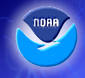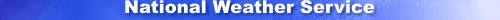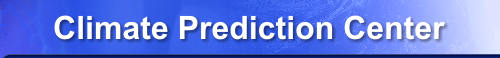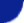home Site Map News Organization SearchCPC Search SM Home United States Soil Moisture Evaporation Precipitation Runoff Temperature OUTLOOK About Us    Our Mission    Who We Are Contact Us    CPC Information    CPC Web TeamHOME > Land Surface Monitoring and PredictionThe outlook and observations are categorized in three equal classes, based on 1961-90 period. We use 102 CDs. The heidke skill score is calculated for the A&B classes combined; we don't issue N forecasts a-priori. The outlook is regarded as falling in the A or B class if the amplitude is half the tercile limit. This is done because the forecast is usually damped, and we want some 60% coverage or so. Even if SS1 were 100, SS2 would be 100*coverage, about 60. (This largely explains why even at negative lead the w30 forecasts (= self specification) do not yield SS2=100.) Definitions: Heidke score - general definition for 3 equal classes SS = 100* (H - E) / (T - E) = 100* (H - T/3) / (T - T/3) = 100*(H - T/3) / (2/3 T) Score on non-CL stations: SS1 = 100 * (H1 - E1) / (T1 - E1) = 100 * (H1 - T1 /3) / (2/3 T1) For a score on all stations, assume that 1/3 of the CL forecasts are right, 2/3 is wrong, i.e. H = H1 + (T-T1)/3. Then: SS2 = (H - T/3) / (2/3 T) *100 = (under above assumption) = SS1 * C where: A (bove), N (ear Normal) ; not used here B (elow) CL =climatological probabilities A, B and N occurred 33.333% of the time during 1961-90. Skill measures the improvement over CL as a 'forecast' everywhere all the time. T = Total # of forecasts = # of stations * # of seasons = 102 for individual month H = # of hits E = # of hits expected by chance = T/3 T1 = Total # of forecasts for non-CL (= combined area of A&B) forecasts H1 = # of hits at non-CL stations E1 = # of hits expected at non-CL stations = T1/3 C = coverage = T1 / T; C*100 is percentage of non-CL stations. NOTE: You don't start to count skill (SS) as positive unless and until more than 1/3rd of the forecasts are categorically correct. 1/3rd is the 'zero' skill level. Pointing at a case (here and there) where your method was right is dangerous, particularly when done after the fact and when SS-overall <=0.
 NOAA/ National Weather Service NOAA Center for Weather and Climate Prediction Climate Prediction Center 5830 University Research Court College Park, Maryland 20740 Page Author: Climate Prediction Center Internet Team Disclaimer Information Quality Credits Glossary Privacy Policy Freedom of Information Act (FOIA) About Us Career Opportunities